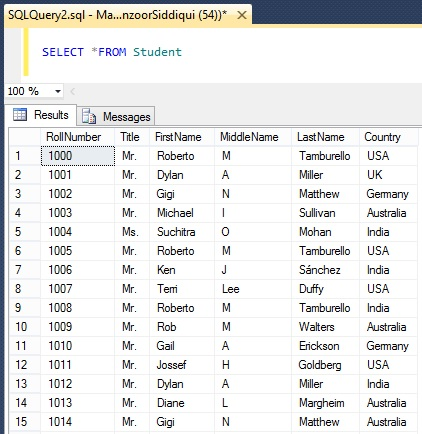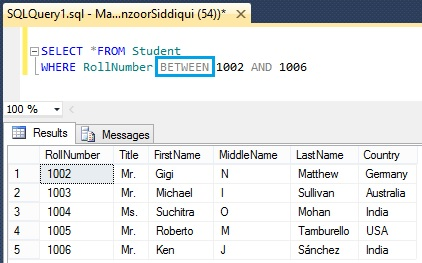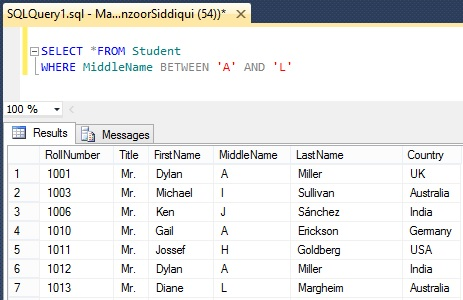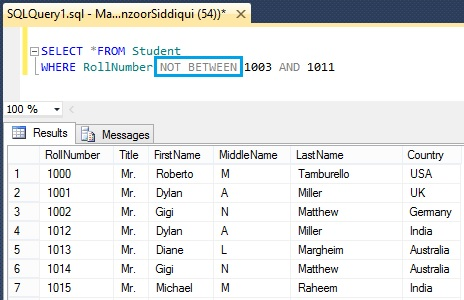# SQL BETWEEN and NOT BETWEEN Operators

The SQL BETWEEN operator retrieves values within a specified range of SELECT, UPDATE, DELETE or INSERT commands and can be numbers, dates or text values. Here we will see examples for BETWEEN and NOT BETWEEN operators.

## Syntax

SELECT column1, column2…, columnN
FROM TableName
WHERE column BETWEEN value1 AND value2

Here we will see how we can use BETWEEN operator from Student table.## Examples

Let’s find RollNumber between 1002 and 1006 from Student table.

```SELECT *FROM Student
WHERE RollNumber BETWEEN 1002 AND 1006```As you can observe, both lower and upper values are inclusive and returns the specified range.

We will see one more example where we will consider values between two characters. Here we will take character ‘A’ and ‘L’ for student middle name.

```SELECT *FROM Student
WHERE MiddleName BETWEEN 'A' AND 'L'```There are seven records for middle name between characters ‘A’ and ‘L’. In the same manner you can also find records between two date range.

If you don’t want records between two specific values then  you can use NOT BETWEEN operator. Here we will see one example.

```SELECT *FROM Student
WHERE RollNumber NOT BETWEEN 1003 AND 1011```• ## SQL NOT BETWEEN Operator

Reference: Manzoor Siddiqui [www.SQLServerLog.com]